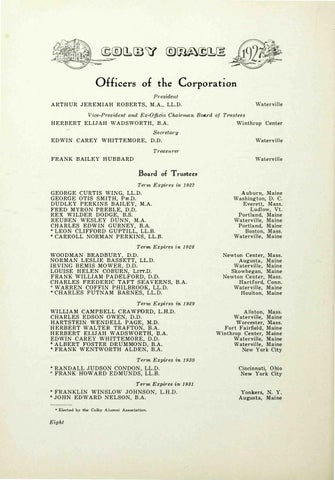Officers of the Corp ora ti on Presidmit Waterville

A RT H U R J E R E M I A H R O B E R T S , M . A . , LL.D.

Vice-Pres id ent and Ex-Officio Chairrnan Boa1·d of Trustees W i nthrop

H E R B E R T E L I J A H W A D S WO R T H , B . A .

Center

Secretary EDWIN

CAREY

WHITTEMORE,

Waterv i l l e

D.D.

Tre.asiirer Waterv i l l e

F R A N K BAILEY H U B B A RD

Board of Trustees Term Expires in

1927

GEO RGE CURTIS W I N G, LL.D. G E O R G E O T I S S M I T H , PH.D. DUDLEY PERKINS BAILEY, M.A. FRED MYRON PREBLE, D.D. R E X W I LD E R D O D G E , B . S . REUBEN WESLEY DUN N , M.A. C H A R L E S E DW I N G U R N E Y , B . A . * L E O N C L I F F O R D G U P T I LL, L L . B . * CARROLL NORMAN PE RKINS, LL.B.

Term Expires in

A u b u r n , M a ine W ashington, D. C. Everett, M ass. Ludlow , V t. Portland, M aine Watervil le, M a ine Portl an d, M aine Boston, M a ss. Watervil le, M a ine 1928

W OO D M A N B R A D B U R Y , D . D . N O R M A N L E S L I E B A S S E T T , LL. D . I R V I N G B E M I S M O W E R , D.D. L O U I S E H E LE N C O B U R N , LITT.D. F RA N K W I L L I A M PA D E L F O R D , D . D . C H A R L E S F R EDE R I C T A F T S E A V E R N S , B . A . * WARREN COFFIN PHI LBROOK, LL.D. * C H A R LES PUTNAM BARNES, LL.D.

Term Expires im

M a ss. M a ine M a ine M a ine M ass. Conn. M a ine M aine

1929

W I L LI A M C A M P B E L L C RA W FO R D , L . H . D . CHARLES EDSON OWEN, D.D. H A R T S T E I N W E N D E L L P A GE , M . D . H E RB E R T W A L T E R T R A F T O N , B . A . H E RB E R T ELIJ A H W A D S W O R T H , B . A . EDWIN CAREY W H I T T E M O R E , D.D. * A L B E R T F O S T E R D R U M MON D , B.A. * F R A N K W E N TW O R T H A L D E N , B.A .

Term Expires im

Newton Center, Augusta , Watervil l e , Skowhegan, Newton Center, H a rtford, Waterville, Houlton,

A l lston, Mass. W aterville, M a ine Worcester, M ass. Fort F a i rfiel d , M aine Winthrop Center, M aine W aterville, M a ine Watervi l le, M a ine New York City 1930

* R A N D A L L J U D S O N CO N DO N , L L . D . * FRANK HOWA R D E D MUN DS, LL.B.

Cincinnati, O hio New York City

Term Expires in 1931 * F R A N K L I N W I N S LOW J O H N S O N , L. H . D . * J O H N E D W A R D N E L S O N , B.A. • Elected by the Colby AJumni Association.

Eight

Yonkers, N. Y . A u gusta, M ai ne